Chemical - Phase - Ionic Equilibriums

# Chemical - Phase - Ionic Equilibriums

Test Description

## 60 Questions MCQ Test Mock Test Series for IIT JAM Chemistry | Chemical - Phase - Ionic Equilibriums

Chemical - Phase - Ionic Equilibriums for Chemistry 2022 is part of Mock Test Series for IIT JAM Chemistry preparation. The Chemical - Phase - Ionic Equilibriums questions and answers have been prepared according to the Chemistry exam syllabus.The Chemical - Phase - Ionic Equilibriums MCQs are made for Chemistry 2022 Exam. Find important definitions, questions, notes, meanings, examples, exercises, MCQs and online tests for Chemical - Phase - Ionic Equilibriums below.
Solutions of Chemical - Phase - Ionic Equilibriums questions in English are available as part of our Mock Test Series for IIT JAM Chemistry for Chemistry & Chemical - Phase - Ionic Equilibriums solutions in Hindi for Mock Test Series for IIT JAM Chemistry course. Download more important topics, notes, lectures and mock test series for Chemistry Exam by signing up for free. Attempt Chemical - Phase - Ionic Equilibriums | 60 questions in 180 minutes | Mock test for Chemistry preparation | Free important questions MCQ to study Mock Test Series for IIT JAM Chemistry for Chemistry Exam | Download free PDF with solutions
 1 Crore+ students have signed up on EduRev. Have you?
Chemical - Phase - Ionic Equilibriums - Question 1

### What amount of solid sodium acetate be added into 1 litre of the 0.1 M CH3COOH solution so that the resulting solution has pH almost equal to pKa (CH3COOH) = 4.74

Detailed Solution for Chemical - Phase - Ionic Equilibriums - Question 1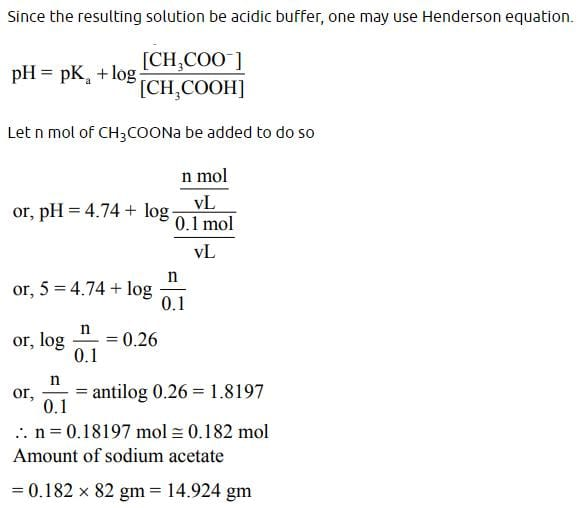Chemical - Phase - Ionic Equilibriums - Question 2

### Liquid ammonia ionizes to a slight extent. At –50°C, its ion product is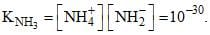How many amide ions, NH-2are present per mm3 of pure liquid ammonia?

Chemical - Phase - Ionic Equilibriums - Question 3

### For preparing a buffer solution of pH 6 by mixing sodium acetate and acetic acid, the ratio of concentration of salt and acid (Ka = 10–5) should be:

Chemical - Phase - Ionic Equilibriums - Question 4

The number of degrees of freedom in the homogenous liquid region of a two component system with a eutectic point, at one atmosphere pressure is:

Chemical - Phase - Ionic Equilibriums - Question 5

The maximum number of triple point(s) occuring in one component sulfur system are:

Chemical - Phase - Ionic Equilibriums - Question 6

Choose correct:

Chemical - Phase - Ionic Equilibriums - Question 7

Consider the following equilibrium in a closed vessel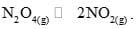. At a fixed temperature if the volume of the reaction vessel is halved, which of the following statements holds true regarding the equilibrium constant (Kp) and the degree of dissociation (α) of N2O4?

Chemical - Phase - Ionic Equilibriums - Question 8

Under conditions of constant temperature and pressure when a system is in equilibrium which of the following quantities is at a minimum:

Detailed Solution for Chemical - Phase - Ionic Equilibriums - Question 8

C is correct.

For a system with controlled constant temperature and pressure, G is minimum at thermodynamic equilibrium.

Chemical - Phase - Ionic Equilibriums - Question 9

Based upon the following hypothetical equilibrium at 273 K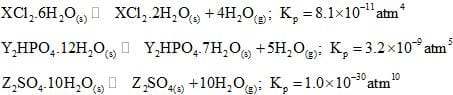Which is the most effective dehydrating agent at 273 K (Aqueous tension at 273 K = 6.0 × 10–3atm)

Chemical - Phase - Ionic Equilibriums - Question 10

An equilibrium system for the reaction between hydrogen and iodine to give hydrogen iodine at 765 K in a 5 liter volume contains 0.4 mole of hydrogen, 0.4 mole of iodine and 2.4 moles of hydrogen iodide. The equilibrium constant for the reaction is: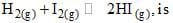Chemical - Phase - Ionic Equilibriums - Question 11

To a 50 ml of 0.1 M HCl solution, 10 ml of 0.1 M NaOH is added and the resulting solution is diluted to 100 ml. What is change in pH of the HCl solution?

Chemical - Phase - Ionic Equilibriums - Question 12

To a 100 ml solution of 0.1 M CH3COONa and 0.1 M CH3COOH, 0.4 gm of solid NaOH was added. Assuming volume remains constant, calculate the change in pH value? Given that pKa(CH3COOH) = 4.74.

Chemical - Phase - Ionic Equilibriums - Question 13

When equal volumes of the following solution are mixed, precipitation of AgCl (Ksp = 1.8 × 10–10) will occur only with:​

Chemical - Phase - Ionic Equilibriums - Question 14

How many gram of CaC2O4 will dissolve in one litre of saturated solution? Ksp of CaC2O4 is 2.5 × 10–9mol–2 and its molecular weight is 128:

Chemical - Phase - Ionic Equilibriums - Question 15

Let the solubilities of AgCl in H2O, 0.01 M CaCl2; 0.01 M NaCl and 0.05 M AgNO3 be S1, S2, S3, S4 respectively. What is the correct relationship between these quantities?

Chemical - Phase - Ionic Equilibriums - Question 16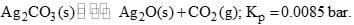Calculate the minimum mole % of CO2 in air at 1 bar which is sufficient to prevent any loss in weight of Ag2CO3 by decomposition at the same temperature:

Chemical - Phase - Ionic Equilibriums - Question 17

At temperature T, a compound AB2(g) dissociates according to the reaction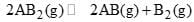with degree of dissociation α, which is small compared with unity. The expression for Kp, in terms of α and the total pressure, PT is:

Chemical - Phase - Ionic Equilibriums - Question 18

A reaction mixture containing H2, N2 and NH3 has partial pressures 2 atm, 1 atm and 3 atm respectively at 725 K. If the value of Kp for the reaction.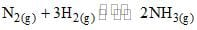is4.28 × 10–5atm–2 at 725 K, in which direction the net reaction will go:

Chemical - Phase - Ionic Equilibriums - Question 19

pH of an aqueous solution of Al+3 is likely to be:

Chemical - Phase - Ionic Equilibriums - Question 20

The molar solubility (in mol L–1) of a sparingly soluble salt MX4 is ‘s’. The corresponding solubility product is Ksp. s is given in terms of Ksp by the relation:

Chemical - Phase - Ionic Equilibriums - Question 21

The phase diagram of a pure substance is sketched below: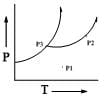The number of degrees of freedom at points P1, P2 and P3, respectively, are:

Chemical - Phase - Ionic Equilibriums - Question 22

Consider a phase diagram of two component simple eutectic system. If XA = 0.8 and XB = 0.2, which of the following composition will never be observed:

Chemical - Phase - Ionic Equilibriums - Question 23

The number of phases, components and degrees of freedom, when Ar is added to an equilibrium mixture of NO, O2 and NO2 in gas phase are, respectively:

Chemical - Phase - Ionic Equilibriums - Question 24

A pure substance exists in several different phases A, B, C. Its partial phase diagram is shown below: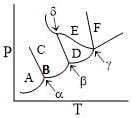Which of the following statements is correct about the above diagram?

Chemical - Phase - Ionic Equilibriums - Question 25

The lowest pressure at which the liquid phase of a pure substance can exist is known as:

Chemical - Phase - Ionic Equilibriums - Question 26

The solid-liquid phase diagram for the Mg-Zn system is shown in the figure below where the vertical line at X(Mg) = 0.33 represents the formation of a congruent melting compound MgZn2.The figure is divided into seven regions depending upon the physical state of the system. The composition of the region #6 represents.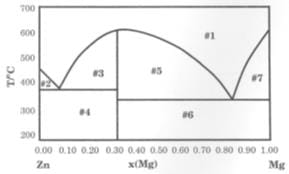Chemical - Phase - Ionic Equilibriums - Question 27

Which of the following does not represent a phase diagram?

Chemical - Phase - Ionic Equilibriums - Question 28

For the reaction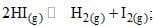the degree of dissociation (α) of HI(g) is related to equilibrium constant Kp by the expression:

Chemical - Phase - Ionic Equilibriums - Question 29

Choose correct:

Chemical - Phase - Ionic Equilibriums - Question 30

Solid CO2 is called ‘dry ice’ because:

*Multiple options can be correct
Chemical - Phase - Ionic Equilibriums - Question 31

pH of the following solution is not affected by dilution:

*Multiple options can be correct
Chemical - Phase - Ionic Equilibriums - Question 32

In the following reaction: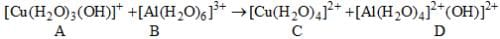*Multiple options can be correct
Chemical - Phase - Ionic Equilibriums - Question 33

When HCl(g) is passed through a saturated solution of common salt, pure NaCl is precipitated because:

*Multiple options can be correct
Chemical - Phase - Ionic Equilibriums - Question 34

Choose the correct statement/s:

*Multiple options can be correct
Chemical - Phase - Ionic Equilibriums - Question 35

Choose correct statement/s:

*Multiple options can be correct
Chemical - Phase - Ionic Equilibriums - Question 36

Formation of Y from X is through the graph. It can be concluded: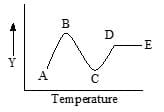*Multiple options can be correct
Chemical - Phase - Ionic Equilibriums - Question 37

The expression for the equilibrium constant for an ideal-gas reaction mixture is given by:

Chemical - Phase - Ionic Equilibriums - Question 38

With a certain radiation (exciting) of a particular frequency, to which hydrogen atoms are exposed, the maximum number of spectral lines is obtainable in the emission is 15. The uppermost energy level to which the is excited is n =

Detailed Solution for Chemical - Phase - Ionic Equilibriums - Question 38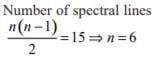*Multiple options can be correct
Chemical - Phase - Ionic Equilibriums - Question 39

Benzene and naphthalene form an ideal solution at room temperature. For this process, the true statement(s) is/are:

*Multiple options can be correct
Chemical - Phase - Ionic Equilibriums - Question 40

Which of the following statements are incorrect:

*Answer can only contain numeric values
Chemical - Phase - Ionic Equilibriums - Question 41

When baking soda is heated in a sealed tube, following equilibrium exists: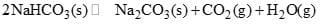If the equilibrium pressure is 1.04 atm at 398 K, calculate the equilibrium constant for the reaction at 398 K [up to two decimal places]:

*Answer can only contain numeric values
Chemical - Phase - Ionic Equilibriums - Question 42

16 moles of H2 and 4 moles of N2 are sealed in a one litre vessel. The vessel is heated at a constant temperature until the equilibrium is established, it is found that the pressure in the vessel has fallen to 9/10 of its original value. Calculate KC for the reaction. [In the multiple of 10–4 up to two decimal places]: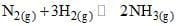*Answer can only contain numeric values
Chemical - Phase - Ionic Equilibriums - Question 43

How many milli-moles of Ca(OH)2 must be dissolved to produce 250 mL of an aqueous solution of pH 10.65, assuming complete dissociation?  [up to two decimal places]

*Answer can only contain numeric values
Chemical - Phase - Ionic Equilibriums - Question 44

For a pure substance, lnP(solid) = –20/T + 10 and lnP(liquid) = –15/T + 2. Calculate enthalpy of fusion. [In the multiple of R]

*Answer can only contain numeric values
Chemical - Phase - Ionic Equilibriums - Question 45

Among CO2, Sulfur, Water, Antimony, Bismuth how many have (dp/dT)fusion is negative?

*Answer can only contain numeric values
Chemical - Phase - Ionic Equilibriums - Question 46

At 0°C, ∆Hfusion(ice) = 1092 Kcal/mol. The molar entropy change for melting of ice at 0°C is? (in kcal/Kmol, up to two decimal places)

*Answer can only contain numeric values
Chemical - Phase - Ionic Equilibriums - Question 47

Find out amount (in gram, up to first decimal place) of NH4Cl dissolved in 500 mL to have pH = 4.5. Kb for NH4OH is 1.8 × 10–5.

*Answer can only contain numeric values
Chemical - Phase - Ionic Equilibriums - Question 48

Calcium lactate is a salt of weak organic acid and strong base, represented as Ca(Lac)2. A saturated solution of Ca(Lac)2 contains 0.13 mole of this salt in 0.50 L solution. The pOH of this solution is 5.60. Assuming a complete dissociation of the salt, calculate Ka of lactic acid. [In the multiple of 10–4, up to first decimal place]

*Answer can only contain numeric values
Chemical - Phase - Ionic Equilibriums - Question 49

The self-ionization constant for pure formic acid, K = [HCOOH2+] [HCOO] has been estimated as 10–6 at room temperature. What percentage of formic acid molecules in pure formic acid are converted to formate ion? The density of formic acid is 1.22 g/cm3. [rounded up to four decimal places]

*Answer can only contain numeric values
Chemical - Phase - Ionic Equilibriums - Question 50

Calculate the pH of solution obtained by mixing 10 mL of 0.1 M HCl and 40 of 0.2 M H2SO4? [up to two decimal places]

*Answer can only contain numeric values
Chemical - Phase - Ionic Equilibriums - Question 51

A sample of HI was found to be 22% dissociated when equilibrium was reached. What will be the degree of dissociated if hydrogen is added in the proportion of 1 mole for every mole of HI originally present, the temperature and volume of the system being kept constant: [up to two decimal places]

*Answer can only contain numeric values
Chemical - Phase - Ionic Equilibriums - Question 52

The pH of pure water at 25°C and 35°C are 7 and 6 respectively. Calculate the heat of formation (cal/mol, up to first decimal place) of water from H+ and OH?

*Answer can only contain numeric values
Chemical - Phase - Ionic Equilibriums - Question 53

Calculate pH change when 0.01 mol CH3COONa solution is added to one litre of 0.01 CH3COOH solution. [up to two decimal places]
Ka (CH3COOH) = 1.8 × 10–5, pKa = 4.74

*Answer can only contain numeric values
Chemical - Phase - Ionic Equilibriums - Question 54

50 mL of 0.1 M NaOH is added to 75 mL of 0.1 M NH4Cl to make a basic buffer. If pKa of NH4+ is 9.26, Calculate pH (up to two decimal places)?

*Answer can only contain numeric values
Chemical - Phase - Ionic Equilibriums - Question 55

Kc for the equilibrium reaction N2O4 dissociating into 2NO2 in chloroform at 291K is 1.14. Calculate free energy change of reaction (Latm) when concentration of two gases are 0.5 mol/L each at same temp. (R = 0.082 LatmK–1mol–1, up to two decimal places)

*Answer can only contain numeric values
Chemical - Phase - Ionic Equilibriums - Question 56

The degree of dissociation at a certain temp of PCl5 at 2 atm is found to be 0.4. At what pressure, the degree of dissociation of PCl5 will be 0.6 at same temp: [up to two decimal places]

*Answer can only contain numeric values
Chemical - Phase - Ionic Equilibriums - Question 57

The vapor density of a mixture containing NO2 and N2O4 is 38.3 at 26.70C. Calculate no of moles of NO2 in 100g mixture: [up to three decimal places]

*Answer can only contain numeric values
Chemical - Phase - Ionic Equilibriums - Question 58

An aqueous system containing K+, Na+, Cl what are number of components if salts are present in equal amount?

*Answer can only contain numeric values
Chemical - Phase - Ionic Equilibriums - Question 59

3 phases are in equilibrium for which T and P can be independently changed at constant composition. Calculate components (C):

*Answer can only contain numeric values
Chemical - Phase - Ionic Equilibriums - Question 60

For a pure substance, lnP(solid) = –20/T + 10 and lnP(liquid) = –15/T + 2. Calculate ratio of slope at triple point [up to two decimal places]:

## Mock Test Series for IIT JAM Chemistry

2 docs|25 tests
 Use Code STAYHOME200 and get INR 200 additional OFF Use Coupon Code
Information about Chemical - Phase - Ionic Equilibriums Page
In this test you can find the Exam questions for Chemical - Phase - Ionic Equilibriums solved & explained in the simplest way possible. Besides giving Questions and answers for Chemical - Phase - Ionic Equilibriums , EduRev gives you an ample number of Online tests for practice

2 docs|25 tests